SSC CGL Previous Year Questions: Algebra- 1

# SSC CGL Previous Year Questions: Algebra- 1 - SSC CGL

Test Description

## 20 Questions MCQ Test SSC CGL (Tier - 1) - Previous Year Papers (Topic Wise) - SSC CGL Previous Year Questions: Algebra- 1

SSC CGL Previous Year Questions: Algebra- 1 for SSC CGL 2023 is part of SSC CGL (Tier - 1) - Previous Year Papers (Topic Wise) preparation. The SSC CGL Previous Year Questions: Algebra- 1 questions and answers have been prepared according to the SSC CGL exam syllabus.The SSC CGL Previous Year Questions: Algebra- 1 MCQs are made for SSC CGL 2023 Exam. Find important definitions, questions, notes, meanings, examples, exercises, MCQs and online tests for SSC CGL Previous Year Questions: Algebra- 1 below.
Solutions of SSC CGL Previous Year Questions: Algebra- 1 questions in English are available as part of our SSC CGL (Tier - 1) - Previous Year Papers (Topic Wise) for SSC CGL & SSC CGL Previous Year Questions: Algebra- 1 solutions in Hindi for SSC CGL (Tier - 1) - Previous Year Papers (Topic Wise) course. Download more important topics, notes, lectures and mock test series for SSC CGL Exam by signing up for free. Attempt SSC CGL Previous Year Questions: Algebra- 1 | 20 questions in 20 minutes | Mock test for SSC CGL preparation | Free important questions MCQ to study SSC CGL (Tier - 1) - Previous Year Papers (Topic Wise) for SSC CGL Exam | Download free PDF with solutions
 1 Crore+ students have signed up on EduRev. Have you?
SSC CGL Previous Year Questions: Algebra- 1 - Question 1

### If (5a – 3b) : (4a – 2b) =2 : 3, then a : b is equal to:       (SSC CGL-2018)

Detailed Solution for SSC CGL Previous Year Questions: Algebra- 1 - Question 1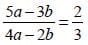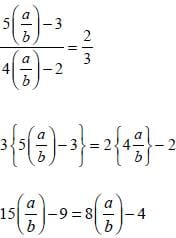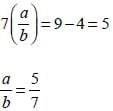SSC CGL Previous Year Questions: Algebra- 1 - Question 2

### If a – b = 5 an d ab = 6, then (a3 – b3) is equal to:        (SSC CGL-2018)

Detailed Solution for SSC CGL Previous Year Questions: Algebra- 1 - Question 2

(a - b)2 = a2 + b2 - 2 ab ⇒ (5)2 = a2 + b2 -2 x 6
∴ a2 + b2 = 25 + 12 = 37
(a3 - b3) = (a - b) (a2 + ab + b2)
= 5 (37 + 6) = 215

SSC CGL Previous Year Questions: Algebra- 1 - Question 3

### If a + b + c = 10 and ab + bc + ca = 32 then a3 + b3 + c3 – 3abc is equal to:       (SSC CGL-2018)

Detailed Solution for SSC CGL Previous Year Questions: Algebra- 1 - Question 3

(a + b + c)2 = a2 + b2 + c2 + 2 (ab + bc + ca)
a2 + b2 + c2 = (a + b + c)2 - 2 (ab + bc + ca)
= (10)2 - 2 (32) = 100 - 64
a2 + b2 + c2 = 36
Now, a3 + b3 + c3 - 3abc
= (a + b + c) (a2 + b2 + c2 - ab - bc - ca)
= 10 (36 - 32) = 40

SSC CGL Previous Year Questions: Algebra- 1 - Question 4

If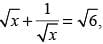then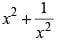is equal to:        (SSC CGL-2018)

Detailed Solution for SSC CGL Previous Year Questions: Algebra- 1 - Question 4

On squaring both sides, we get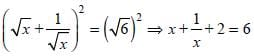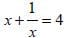Again, squaring on both sides,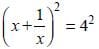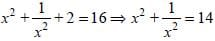SSC CGL Previous Year Questions: Algebra- 1 - Question 5

If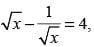then x2 + (1/x2) is equal to:        (SSC CGL-2018)

Detailed Solution for SSC CGL Previous Year Questions: Algebra- 1 - Question 5

Given that: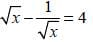Squaring both sides, we get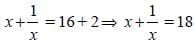Again, squaring both sides, we get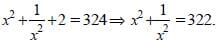SSC CGL Previous Year Questions: Algebra- 1 - Question 6

If a : b = 3 : 2, then (5a + 2b) : (3a + 4b) is equal to:        (SSC CGL-2018)

Detailed Solution for SSC CGL Previous Year Questions: Algebra- 1 - Question 6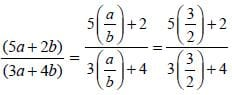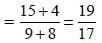SSC CGL Previous Year Questions: Algebra- 1 - Question 7

If a + b + c = 13 and ab + bc + ca = 54, then a3 + b3 + c3 – 3abc is equal to:       (SSC CGL-2018)

Detailed Solution for SSC CGL Previous Year Questions: Algebra- 1 - Question 7

(a + b + c)2 = a2 + b2 + c2 + 2(ab + bc + ca)
(13)2 = a2 + b2 + c2 + 2(54)
a2 + b2 + c2 = 169 – 108 = 61
Now, a3 + b3 + c3 – 3abc = (a + b + c) (a2 + b2 + c2 – ab – bc – ca)
= 13(61 – 54) = 13 x 7 = 91

SSC CGL Previous Year Questions: Algebra- 1 - Question 8

If x + (1/x)= 3, then x3 + (1/(x3)) is equal to:       (SSC CGL-2018)

Detailed Solution for SSC CGL Previous Year Questions: Algebra- 1 - Question 8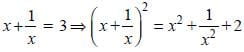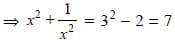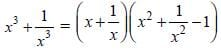= 3 (7 – 1) = 3 x 6 = 18

SSC CGL Previous Year Questions: Algebra- 1 - Question 9

If a2 + b2 = 169, ab = 60, (a > b), then (a2 – b2) is equal to :       (SSC CHSL-2018)

Detailed Solution for SSC CGL Previous Year Questions: Algebra- 1 - Question 9

From question,
(a + b)2 = a2 + b2 + 2ab
= 169 + 2 × 60
(a + b)2 = 289 ⇒ (a + b) = 17 ...(i)
and
(a – b)2 = a2 + b2 – 2ab
= 169 – 120 = 49
(a – b) = √49 = 7 ...(ii)
from (i) and (ii), we get
(a + b) (a – b) = 17 × 7
a2 – b2 = 119

SSC CGL Previous Year Questions: Algebra- 1 - Question 10

If (2x + 3)3 + (x – 8)3 + (x + 13)3 = (2x + 3) (3x – 24) (x +13), then what is the value of x?       (SSC Sub. Ins. 2018 )

Detailed Solution for SSC CGL Previous Year Questions: Algebra- 1 - Question 10

(2x + 3)3 + (x – 8)3 + (x + 13)3 = (2x + 3) (3x – 24) (x + 13)
(2x + 3)3 + (x – 8)3 + (x + 13)3 = 3(2x + 3)(x – 8)(x +13)
We know that if a3 + b3 + c3 = 3 abc
then, a + b + c = 0
(2x + 3) + (x – 8) + (x + 13) = 0
4x + 8 = 0
x = (-8)/4
∴ x = – 2

SSC CGL Previous Year Questions: Algebra- 1 - Question 11

If a3 + b3 = 5824 and a + b = 28, then (a – b)2 + ab is equal to:         (SSC Sub. Ins. 2018 )

Detailed Solution for SSC CGL Previous Year Questions: Algebra- 1 - Question 11

a3 + b3 = 5824
(a + b) (a2 + b2 – ab) = 5824
28(a2 + b2 – ab) = 5824
(a2 + b2 – ab) = 5824/28 = 208
Now, (a – b)2 + ab = a2 + b2 – 2ab + ab
= a2 + b2 – ab = 208

SSC CGL Previous Year Questions: Algebra- 1 - Question 12

If x – (1/x) = 6, then x3 – (1/x3) is equal to:       (SSC Sub. Ins. 2018 )

Detailed Solution for SSC CGL Previous Year Questions: Algebra- 1 - Question 12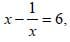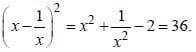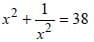Now.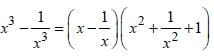= 6(38 + 1) = 6 × 39 = 234.

SSC CGL Previous Year Questions: Algebra- 1 - Question 13

If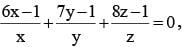then what is the value of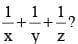(SSC Sub. Ins. 2017)

Detailed Solution for SSC CGL Previous Year Questions: Algebra- 1 - Question 13

Here,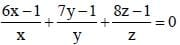Then,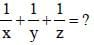Now,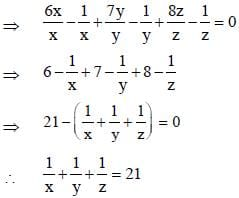SSC CGL Previous Year Questions: Algebra- 1 - Question 14

If x2 – 3x + 1 = 0, then what is the value of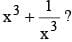(SSC Sub. Ins. 2017)

Detailed Solution for SSC CGL Previous Year Questions: Algebra- 1 - Question 14

If x2 – 3x + 1 = 0 then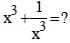Dividing equation by x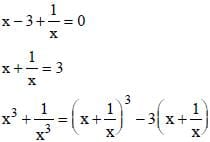= (3)3 – 3 × 3
= 27 – 9 = 18

SSC CGL Previous Year Questions: Algebra- 1 - Question 15

If a = 2017, b = 2016 and c = 2015, then what is the value of a2 + b2 + c2 – ab – bc – ca?    (SSC Sub. Ins. 2017)

Detailed Solution for SSC CGL Previous Year Questions: Algebra- 1 - Question 15

Here, a = 2017, b = 2016, and c = 2015
∴ a2 + b2 + c2 – ab – bc – ca
⇒ (2017)2 + (2016)2 + (2015)2 – 2017× 2016 – 2016 × 2015 – 2015 × 2017 = 3

SSC CGL Previous Year Questions: Algebra- 1 - Question 16

If the expression (px3 – 8x2 – qx + 1) is completely divisible by the expression (3x2 – 4x + 1), then what will be the value of p and q respectively?          (SSC Sub. Ins. 2017)

Detailed Solution for SSC CGL Previous Year Questions: Algebra- 1 - Question 16

Let p(x) = px3 – 8x2 – qx + 1
Since, (3x2 – 4x + 1) is factor of p (x), so p (a) = 0
∴ 3x2 – 4x + 1 = 0
3x2 – 3x – x + 1 = 0
3x (x – 1) – 1 (x – 1) = 0
(3x – 1) (x – 1) = 0
∴ x = 1/3, 1
∴ p(x) = 1/3, 1
i.e.,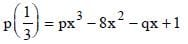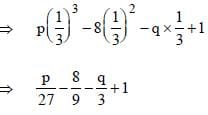⇒ p – 24 – 9q + 27
⇒ p – 9q = –3 ...(i)
p (1) = px3 – 8x2 – qx + 1
⇒ p (1)3 – 8 (1)2 – q × 1 + 1
⇒ p – 8 – q + 1
⇒ p – q = 7 ....(ii)
From Eq. (i) and (ii),
p = 33/4, q = 5/4

SSC CGL Previous Year Questions: Algebra- 1 - Question 17

If (p2 + q2) / (r2 + s2) = (pq) / (rs), then what is the value of (p – q) (p + q) in terms of r and s?       (SSC Sub. Ins. 2017)

SSC CGL Previous Year Questions: Algebra- 1 - Question 18

If 'a' and 'b' are positive integers such that a2 – b2 = 19, then the value of 'a' is :       (SSC MTS 2017)

Detailed Solution for SSC CGL Previous Year Questions: Algebra- 1 - Question 18

According to question,
a2 – b2 = 19
(a + b) (a – b) = 19
Since 19 is prime, one of (a + b) (a – b) is 19
Therefore, (10)2 – (9)2 = 19
∴ a = 10

SSC CGL Previous Year Questions: Algebra- 1 - Question 19

The line passing through (–2, 5) and (6, b) is perpendicular to the line 20x + 5y = 3. Find b?       (SSC CHSL 2017)

Detailed Solution for SSC CGL Previous Year Questions: Algebra- 1 - Question 19

Here,
20x + 5y = 3
⇒ 5y = – 20x + 3
∴ y = – 4x + (3/5)
Slope of 20x + 5y = 3 ⇒ –4
We know, product of slopes = –1 for perpendicular lines
Hence, the slope of the line which passes through (–2, 5) and (6, b) =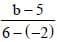Now,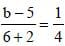⇒ b – 5 = 2
∴ b = 5 + 2 = 7

SSC CGL Previous Year Questions: Algebra- 1 - Question 20

If x – y = 6 and xy = 40, then find x2 + y2?       (SSC CHSL 2017)

Detailed Solution for SSC CGL Previous Year Questions: Algebra- 1 - Question 20

Here,
x – y = 6, xy = 40, x2 + y2 = ?
(x – y)2 = (6)2
x2 + y2 – 2. x. y = 36
∴ x2 + y2 = 36 + 2xy
⇒ 36 + 2 × 40
= 116

## SSC CGL (Tier - 1) - Previous Year Papers (Topic Wise)

250 tests
Information about SSC CGL Previous Year Questions: Algebra- 1 Page
In this test you can find the Exam questions for SSC CGL Previous Year Questions: Algebra- 1 solved & explained in the simplest way possible. Besides giving Questions and answers for SSC CGL Previous Year Questions: Algebra- 1, EduRev gives you an ample number of Online tests for practice

250 tests